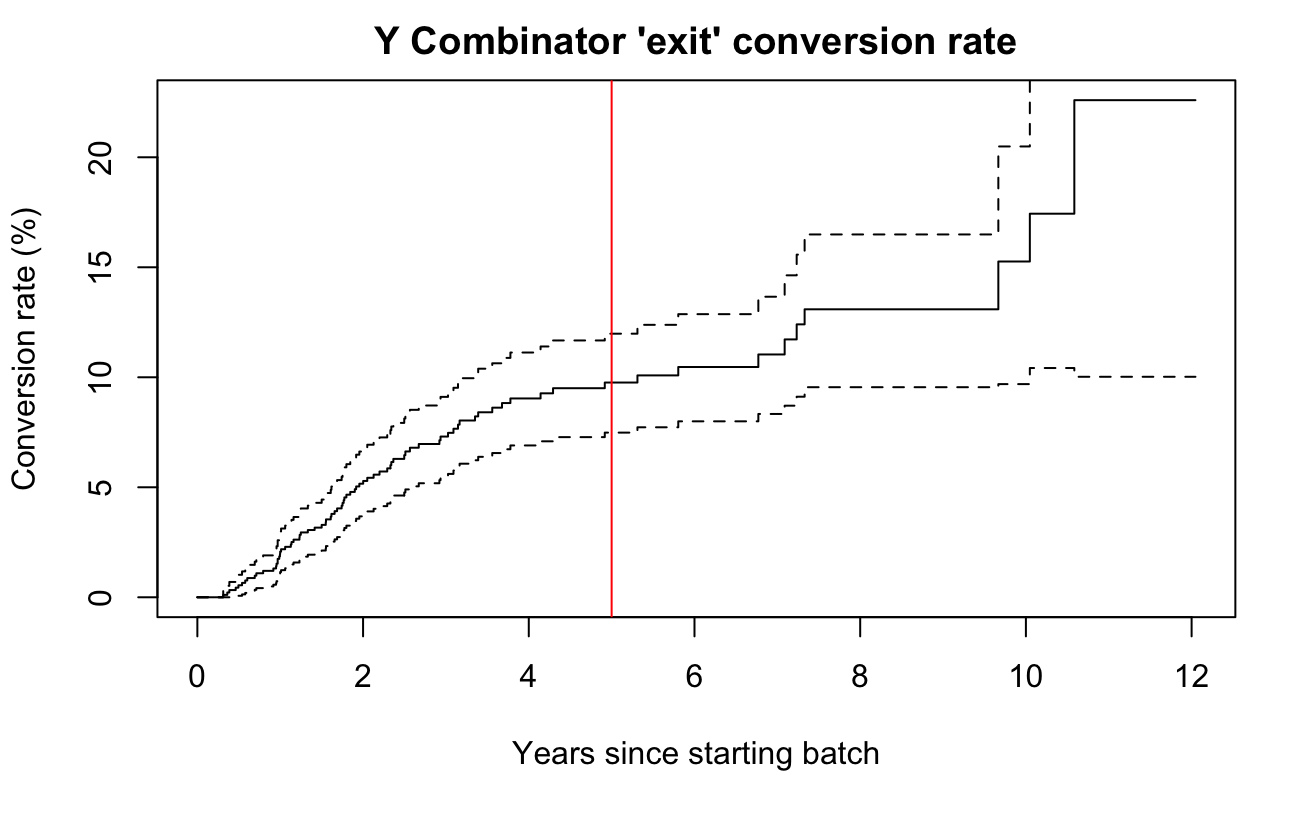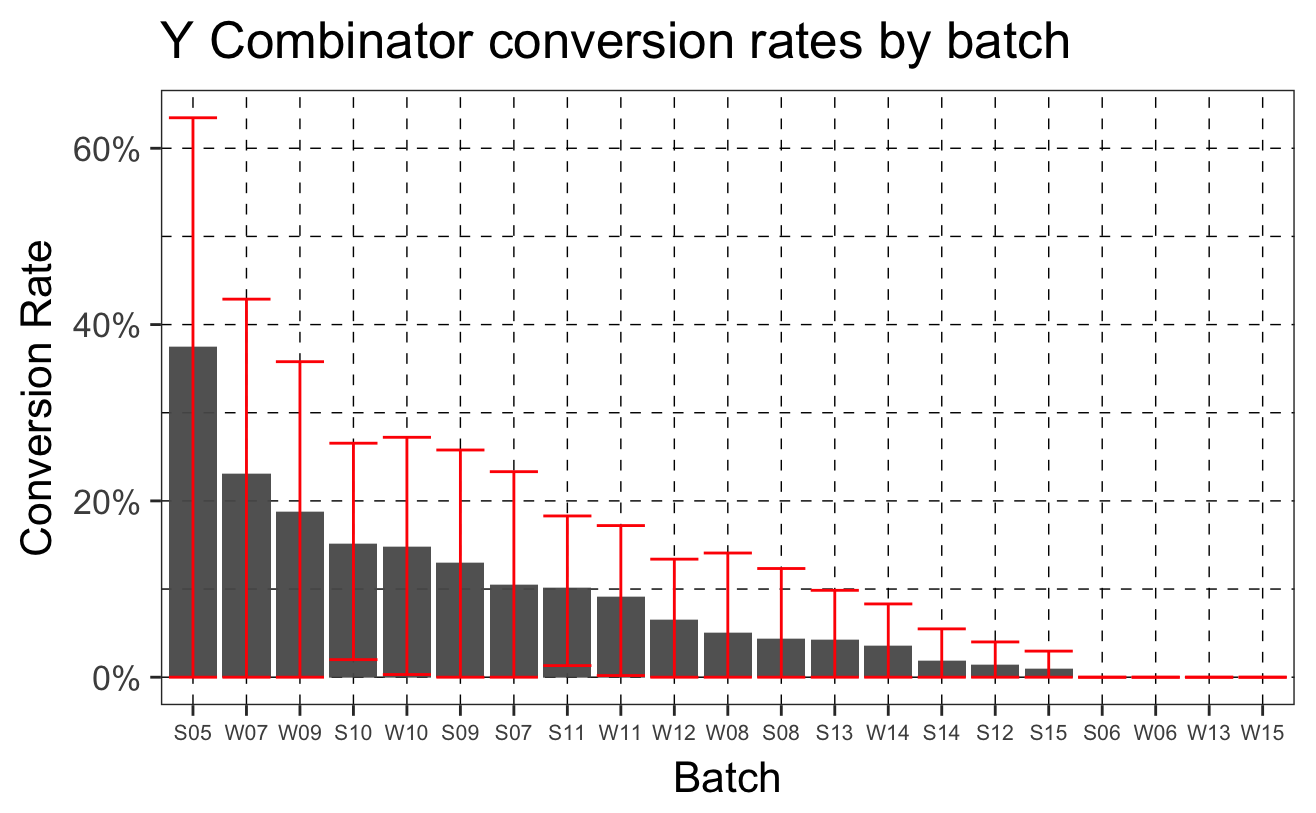A few months ago, I was walking around Mountain View while chatting with Zapier’s Co-founder Bryan. I wondered, “what’s Y Combinator’s conversion rate?” He didn’t know but suggested yclist.com as a place I might find data useful to answer the question.

``````Sys.setenv(TZ='GMT')
library(rvest) # for web scraping
library(dplyr) # for manipulating data
library(readr) # for reading data from a csv that I'll use as a data entry tool
library(survival) # R's basic survival anaylsis toolkit
library(ggplot2) # R plotting library
library(purrr) # functional programming library
library(ggthemes) # library with themes for ggplot2``````
``````site <- read_html("http://yclist.com/")
extract <- function(data, xpath) {
data %>%
html_nodes(xpath = xpath) %>%
html_text()
}
companies <- tibble::tibble(
company = site %>% extract(
"//td[(((count(preceding-sibling::*) + 1) = 2) and parent::*)]"),
batch = site %>% extract(
"//td[(((count(preceding-sibling::*) + 1) = 4) and parent::*)]") %>%
gsub("\n", "", .) %>%
gsub(" ", "", .),
status = site %>% extract(
"//td[(((count(preceding-sibling::*) + 1) = 5) and parent::*)]")
)
start_date <- function(batch) {
if(!grepl("W", batch) & !grepl("S", batch)) return(NA)
month <- ifelse(grepl("W", batch), "01", "06")
year <- batch %>% gsub("W", "", .) %>%
gsub("S", "", .) %>%
paste0("20", .)
paste0(year, "-", month, "-01") %>%
as.POSIXct(origin = "1970-01-01", tz = "UTC")
}
# I read out the data as a .csv, added my own column and hand-by-hand used Crunchbase and Google to find exit dates. Please let me know if you see any that are wrong!
# companies %>%
#   mutate(start = as.POSIXct(Vectorize(start_date)(batch), origin = "1970-01-01")) %>%
#   filter(status == "Exited") %>%
#   select(company) %>%
#   write.csv("exits.csv", row.names = FALSE)
outcomes <- companies %>%
mutate(start = as.POSIXct(Vectorize(start_date)(batch), origin = "1970-01-01")) %>%
filter(!grepl("F", batch)) %>%
left_join(
read.csv("~/yc/exits.csv", stringsAsFactors = FALSE) %>% tbl_df(),
by = "company"
) %>%
mutate(time = ifelse(
is.na(exit), difftime(Sys.time(), start, units = "days"),
difftime(exit, start, units = "days")),
censor = ifelse(!is.na(exit) | status == "Exited", 1, 0)
)``````
``````outcomes %>%
survfit(Surv(time, censor) ~ 1, data = .) %>%
plot(fun = "event", xscale=365.25,
main = "Y Combinator 'exit' conversion rate",
xlab = "Years since starting batch",
yscale = 100,
ylab = "Conversion rate (%)")
abline(v = 5*365.25, col = 'red')``````One in twenty YC startups exited within two years of starting the batch. That grows to one in ten by year five.

``````outcomes %>%
survfit(Surv(time, censor) ~ 1, data = .) %>%
summary(times = seq_len(12)*365.25) %>%
{ tibble::tibble(time = .\$time, conv_rate = 1 - .\$surv) } %>%
mutate(`Marginal Conv` = conv_rate - lag(conv_rate)) %>%
mutate(conv_rate = scales::percent(conv_rate),
`Marginal Conv` = ifelse(is.na(`Marginal Conv`), NA, scales::percent(`Marginal Conv`))) %>%
mutate(time = time / 365.25) %>%
rename(`Years` = time, `Conv. Rate` = conv_rate)``````

I thought it’d be interesting to compare batches by two-year conversion rate,

``````outcomes %>%
survfit(Surv(time, censor) ~ batch, data = .) %>%
summary(times = 365.25*2) %>%
{ tibble::tibble(batch = as.character(.\$strata),
lower = 1 - .\$upper,
conv_rate = 1 - .\$surv,
upper = 1 - .\$lower) } %>%
mutate(batch = gsub("batch=", "", batch)) %>%
arrange(desc(conv_rate)) %>%
mutate(batch = factor(batch, levels = batch)) %>%
ggplot(aes(x = batch)) +
geom_bar(stat = "identity",
aes(y = conv_rate),
alpha = 0.95) +
geom_errorbar(aes(ymin = lower, ymax = upper), colour = 'red') +
theme_bw(base_size = 16) +
scale_y_continuous(labels = scales::percent) +
theme(panel.grid.major = element_line(size = 0.25, linetype = "dashed", colour = "black"),
panel.grid.minor = element_line(size = 0.25, linetype = "dashed", colour = "black"),
axis.text.x = element_text(size = 8)) +
xlab("Batch") +
ylab("Conversion Rate") +
ggtitle("Y Combinator conversion rates by batch")``````At a cursory glance, it appears earlier batches had a higher conversion rates. This is confirmed by including one through four years post-batch.

``````outcomes %>%
mutate(vintage = format(start, "%Y")) %>%
survfit(Surv(time, censor) ~ vintage, data = .) %>%
summary(times = seq_len(4)*365.25) %>%
{ tibble::tibble(year = .\$time / 365.25,
vintage = as.character(.\$strata),
lower = 1 - .\$upper,
conv_rate = 1 - .\$surv,
upper = 1 - .\$lower) } %>%
mutate(vintage = gsub("vintage=", "", vintage)) %>%
arrange(desc(conv_rate)) %>%
ggplot(aes(x = vintage, fill = factor(year))) +
geom_bar(stat = "identity",
position = "dodge",
aes(y = conv_rate),
alpha = 0.95) +
theme_bw(base_size = 16) +
scale_y_continuous(labels = scales::percent) +
scale_fill_colorblind(name = "Years since batch") +
theme(panel.grid.major = element_line(size = 0.25, linetype = "dashed", colour = "black"),
panel.grid.minor = element_line(size = 0.25, linetype = "dashed", colour = "black"),
axis.text.x = element_text(size = 8),
legend.position = "top") +
xlab("Vintage") +
ylab("Conversion Rate") +
ggtitle("Y Combinator conversion rates by vintage")``````